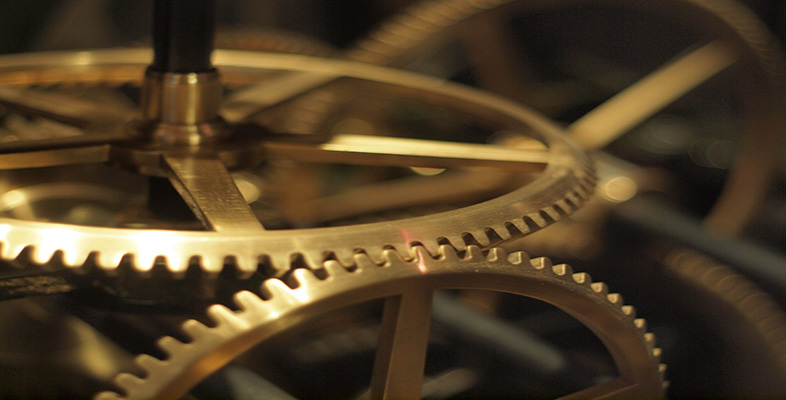Digital & Computing

### Become an OU studentCrossing the boundary: analogue universe, digital worlds

Start this free course now. Just create an account and sign in. Enrol and complete the course for a free statement of participation or digital badge if available.

# 3.9 A few more terms

Just to round off this description of the interior of the digital world, let me introduce and define a few more terms that you will come across again in this course and in any future studies of computers. Specifically, you may have heard the terms bits, bytes and words used in connection with computers. Now that we have taken a look at the binary system that underlies computer arithmetic, you will find there is no mystery in any of these three terms.

The word Bit is short for binary digit and refers to a 1 or a 0 stored in the computer. Since all computers have some limit to the size of their memory there are only so many bits that a particular computer can store.

A byte is a group of a certain number of bits, usually eight. Now, if we take the eight bits together, and represent a byte pictorially, it will look like this:

bit 8   bit 7   bit 6   bit 5   bit 4   bit 3   bit 2   bit 1

Recall that each bit, or binary digit, can be either 1 or 0. This means we can think of the byte as representing a binary number. Therefore the largest number we can store in the eight bits of a byte is:

bit 8   bit 7   bit 6   bit 5   bit 4   bit 3   bit 2   bit 1
11111111

And the smallest number is:

bit 8   bit 7   bit 6   bit 5   bit 4   bit 3   bit 2   bit 1
00000000

## Exercise 7

What do the binary numbers 0000000 and 11111111 represent in decimal?

### Discussion

00000000 = decimal 0

11111111 = decimal 255 (one group of 128, plus one group of sixty-four, plus one group of thirty-two, plus one group of sixteen, plus one group of eight, plus one group of four, plus one group of two plus one)

A word is generally a group of four bytes. It is the largest data object a particular computer can process in a single operation.

In the scheme we have discussed, a word is 32 bits (4×8), so the largest binary number that a computer using a four-byte word can process in a single operation would be

11111111111111111111111111111111

which is decimal 4,294,967,295. (In fact computers can handle much larger numbers than this, but need extra software support to do so.

You will probably have noticed that the size of your computer's memory and hard drive is measured in bytes. But memories are so large now that it is impractical to count single bytes. A few years ago, memory size was usually rated in kilobytes (KBs), that is, thousands of bytes. Now it is measured in megabytes (MBs) – millions of bytes, or even gigabytes (GBs) – thousands of millions of bytes). The computer on which I am writing this course has a memory of 512MB, or 512 million bytes. Drive sizes are even larger, so a typical hard drive will now hold 80–100GB, 80–100 thousand million bytes.

But beware. There is confusion here. When computer scientists talk about a kilobyte, or a kilo- anything, they don't strictly mean one thousand. A computer kilo- is actually 1024 and a computer mega- is 1,048,576.

## SAQ 5

Why do you think computer scientists use these strange values, rather than a simple 1,000 and 1,000,000?